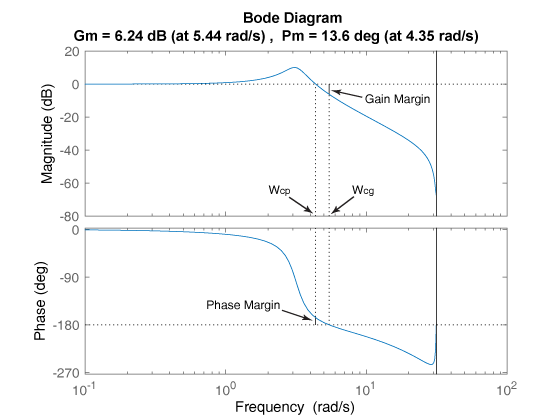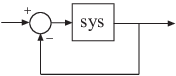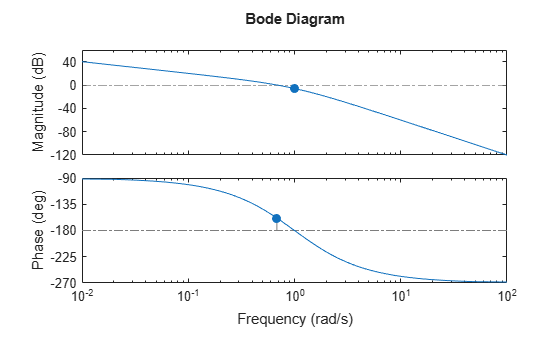Documentation

### This is machine translation

Mouseover text to see original. Click the button below to return to the English version of the page.

# margin

Gain margin, phase margin, and crossover frequencies

## Syntax

``margin(sys)``
``[Gm,Pm,Wcg,Wcp] = margin(sys)``
``[Gm,Pm,Wcg,Wcp] = margin(mag,phase,w)``
``[Gm,Pm] = margin(sys,J1,...,JN)``

## Description

example

````margin(sys)` plots the Bode response of `sys` on the screen and indicates the gain and phase margins on the plot. Gain margins are expressed in dB on the plot.Solid vertical lines mark the gain margin and phase margin. The dashed vertical lines indicate the locations of `Wcp`, the frequency where the phase margin is measured, and `Wcg`, the frequency where the gain margin is measured. The plot title includes the magnitude and location of the gain and phase margin.`Gm` and `Pm` of a system indicate the relative stability of the closed-loop system formed by applying unit negative feedback to `sys`, as shown in the following figure.`Gm` is the amount of gain variance required to make the loop gain unity at the frequency `Wcg` where the phase angle is –180° (modulo 360°). In other words, the gain margin is 1/g if g is the gain at the –180° phase frequency. Similarly, the phase margin is the difference between the phase of the response and –180° when the loop gain is 1.0. The frequency `Wcp` at which the magnitude is 1.0 is called the unity-gain frequency or gain crossover frequency. Usually, gain margins of three or more combined with phase margins between 30° and 60° result in reasonable tradeoffs between bandwidth and stability.```

example

````[Gm,Pm,Wcg,Wcp] = margin(sys)` returns the gain margin `Gm` in absolute units, the phase margin `Pm`, and the corresponding frequencies `Wcg` and `Wcp`, of `sys`. `Wcg` is the frequency where the gain margin is measured, which is a –180° phase crossing frequency. `Wcp` is the frequency where the phase margin is measured, which is a 0-dB gain crossing frequency. These frequencies are expressed in radians/`TimeUnit`, where `TimeUnit` is the unit specified in the `TimeUnit` property of `sys`. When `sys` has several crossovers, `margin` returns the smallest gain and phase margins and corresponding frequencies.```

example

````[Gm,Pm,Wcg,Wcp] = margin(mag,phase,w)` derives the gain and phase margins from frequency response data. Provide the gain data `mag` in absolute units, and phase data `phase` in degrees. You can provide the frequency vector `w` in any units and `margin` returns `Wcg` and `Wcp` in the same units.```

example

````[Gm,Pm] = margin(sys,J1,...,JN)` returns the gain margin `Gm` and phase margin `Pm` of the entries in model array `sys` with subscripts `(J1,...,JN)`.```

## Examples

collapse all

For this example, create a continuous transfer function.

`sys = tf(1,[1 2 1 0])`
```sys = 1 --------------- s^3 + 2 s^2 + s Continuous-time transfer function. ```

Display the gain and phase margins graphically.

`margin(sys)`The gain margin (6.02 dB) and phase margin (21.4 deg), displayed in the title, are marked with solid vertical lines. The dashed vertical lines indicate the locations of `Wcg`, the frequency where the gain margin is measured, and `Wcp`, the frequency where the phase margin is measured.

For this example, create a discrete-time transfer function.

`sys = tf([0.04798 0.0464],[1 -1.81 0.9048],0.1)`
```sys = 0.04798 z + 0.0464 --------------------- z^2 - 1.81 z + 0.9048 Sample time: 0.1 seconds Discrete-time transfer function. ```

Compute the gain margin, phase margin and frequencies.

`[Gm,Pm,Wcg,Wcp] = margin(sys)`
```Gm = 2.0517 ```
```Pm = 13.5711 ```
```Wcg = 5.4374 ```
```Wcp = 4.3544 ```

The results indicate that a gain variation of over 2.05 dB at the gain crossover frequency of 5.43 rad/s would cause the system to be unstable. Similarly a phase variation of over 13.57 degrees at the phase crossover frequency of 4.35 rad/s will cause the system to lose stability.

For this example, load the frequency response data of an open loop system, consisting of magnitudes (m) and phase values (p) measured at the frequencies in w.

`load('openLoopFRD.mat','p','m','w');`

Compute the gain and phase margins.

`[Gm,Pm,Wcg,Wcp] = margin(m,p,w)`
```Gm = 0.6249 ```
```Pm = 48.9853 ```
```Wcg = 1.2732 ```
```Wcp = 1.5197 ```

For this example, load `invertedPendulumArray.mat`, which contains a 3-by-3 array of inverted pendulum models. The mass of the pendulum varies as you move from model to model along a single column of `sys`, and the length of the pendulum varies as you move along a single row. The mass values used are 100g, 200g and 300g, and the pendulum lengths used are 3m, 2m and 1m respectively.

`$\begin{array}{cccc}& Column\phantom{\rule{0.5em}{0ex}}1& Column\phantom{\rule{0.5em}{0ex}}2& Column\phantom{\rule{0.5em}{0ex}}3\\ Row\phantom{\rule{0.5em}{0ex}}1& 100g,3m& 100g,2m& 100g,1m\\ Row\phantom{\rule{0.5em}{0ex}}2& 200g,3m& 200g,2m& 200g,1m\\ Row\phantom{\rule{0.5em}{0ex}}3& 300g,3m& 300g,2m& 300g,1m\end{array}$`

```load('invertedPendulumArray.mat','sys'); size(sys)```
```3x3 array of transfer functions. Each model has 1 outputs and 1 inputs. ```

Find gain and phase margin for all models in the array.

`[Gm,Pm] = margin(sys)`
```Gm = 3×3 0.9800 0.9800 0.9800 0.9800 0.9800 0.9800 0.9800 0.9800 0.9800 ```
```Pm = 3×3 -11.3565 -11.3898 -11.4228 -11.3842 -11.4088 -11.4333 -11.4020 -11.4208 -11.4396 ```

`margin` returns two arrays, `Gm` and `Pm`, in which each entry is the gain and phase margin values of the corresponding entry in `sys`. For instance, the gain and phase margin of the model with 100g pendulum weight and 2m length is `Gm(1,2)` and `Pm(1,2)`, respectively.

## Input Arguments

collapse all

Dynamic system, specified as a SISO dynamic system model, or an array of SISO dynamic system models. Dynamic systems that you can use include continuous-time or discrete-time numeric LTI models such as `tf`, `zpk`, or `ss` models.

If `sys` is a generalized state-space model `genss` or an uncertain state-space model `uss`, `margin` returns the gain margin and phase margin of the current or nominal value of `sys`. If `sys` is an array of models, `margin` returns the `Gm` and `Pm` of the model corresponding to its subscript `J1,...,JN` in `sys`. For more information on model arrays, see Model Arrays.

Indices of models in array whose gain and phase margins you want to extract, specified as positive integers. You can provide as many indices as there are array dimensions in `sys`. For example, if `sys` is a 4-by-5 array of dynamic system models, the following command extracts `Gm` and `Pm` for entry (2,3) in the array.

`[Gm,Pm] = margin(sys,2,3);`

Magnitude of the system response in absolute units, specified as a 3-D array. Since `margin` only accepts SISO systems, `mag` is a 1-by-1-by-N array, where N is the number of frequency points. For an example, see Obtain Magnitude and Phase Data.

Phase of the system response in degrees, specified as a 3-D array. Since `margin` only accepts SISO systems, `phase` is a 1-by-1-by-N array, where N is the number of frequency points. For an example, see Obtain Magnitude and Phase Data.

Frequencies at which the magnitude and phase values of system response are obtained, specified as a column vector.

## Output Arguments

collapse all

Gain margin, returned as a scalar or an array. If `sys` is:

• A single model, then `Gm` is returned as a scalar.

• A model array, then `Gm` is an array of gain margins of each model in `sys`.

`Gm` is the amount of gain variance required to make the loop gain unity at the frequency `Wcg` where the phase angle is –180° (modulo 360°). In other words, the gain margin is 1/g if g is the gain at the –180° phase frequency. Negative gain margins indicate that stability is lost by decreasing the gain, while positive gain margins indicate that stability is lost by increasing the gain

The gain margin `Gm` is computed in absolute units. You can compute the gain margin in dB by,

```Gm_dB = 20*log10(Gm) ```

Phase margin, returned as a scalar or an array. If `sys` is:

• A single model, then `Pm` is returned as a scalar.

• A model array, then `Pm` is an array of phase margins of each model in `sys`.

The phase margin is the difference between the phase of the response and –180° when the loop gain is 1.0.

The phase margin `Pm` is expressed in degrees.

Gain crossover frequency, returned as a scalar. `Wcg` is the frequency where the gain margin is measured, which is a –180° phase crossing frequency.

`Wcg` is expressed in radians/`TimeUnit`, where `TimeUnit` is the unit specified in the `TimeUnit` property of `sys`.

Phase crossover frequency, returned as a scalar. `Wcp` is the frequency where the phase margin is measured, which is a 0-dB gain crossing frequency.

`Wcp` is expressed in radians/`TimeUnit`, where `TimeUnit` is the unit specified in the `TimeUnit` property of `sys`.

## Tips

• When you use `margin(mag,phase,w)`, `margin` relies on interpolation to approximate the margins, which generally produce less accurate results. For example, if there is no 0-dB crossing within the `w` range, `margin` returns a phase margin of `Inf`. Therefore, if you have an analytical model `sys`, using ```[Gm,Pm,Wcg,Wcp] = margin(sys)``` is a more robust way to obtain the margins.